Search IntMath
Close

450+ Math Lessons written by Math Professors and Teachers

5 Million+ Students Helped Each Year

1200+ Articles Written by Math Educators and Enthusiasts

Simplifying and Teaching Math for Over 23 Years

#### Tips, tricks, lessons, and tutoring to help reduce test anxiety and move to the top of the class.

By Kathleen Cantor, 03 Jul 2020

Before we begin simplifying radical expressions, let’s recall the properties of them. Take a look at the expression below: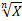Looking at the radical expression above, we can determine that is the radicand of the expression. Meanwhile, √ is the radical symbol while n is the index. In this case, should you encounter a radical expression that is written like this: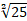You can easily tell that the radicand of the expression equals to 25 while the index equals to 2. Radical expressions with the index of 2 are also referred to as square root. Since that is the case, we can hide the index part so it would be written like this: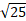## Basics of Simplifying Expressions

If we want to simplify the expression above, we can do it like so: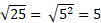Simplifying an expression meaning we are replacing it with an equivalent that is easier to digest and, if possible, shorter. In the example above, the simplification of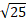​ is 5. If we want to simplify other radicals such as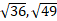​, and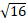​ that has perfect square radicands—25 is also a perfect square, then the result would be 6, 7, and 4 respectively.

Recall that perfect squares are radicands that have an integer as its square root (e.g. 5, an integer, is the square root of 25). In that case, what if we want to simplify other radicals that don’t have a perfect square as its radicands?

Imperfect squares are the opposite of perfect squares. As radicands, imperfect squares don’t have an integer as its square root. Instead, the square root would be a number which decimal part would continue on endlessly without end and won’t show any repeating pattern. Here are some examples:

•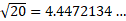•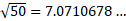•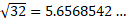As you can see, the decimal part of these square roots won’t repeat nor terminate. These numbers can’t even be expressed accurately with fractions of integers. How do we simplify them? To tell you the truth, it’s quite simple.

•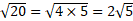•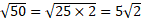•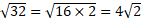How did we do it? Well, in reality, there’s another property of radical expressions, which is the Product Rule of radical expressions.

### The Product Rule of Radical Expressions

The Product Rule indicates radical expression behavior. That is, if two or more radical expressions with the same index—let’s say n—are multiplied, the result would be equal to the radical expression of the product between the previous radicands, with the index of n. To understand it better, consider the equation below: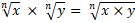With this rule, we can more easily simplify radical expressions that are seemingly complicated like before. Let’s deepen our understanding with a few more examples.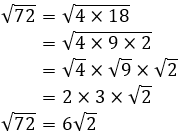From the equation above, we separate the radicand (72) into three different numbers (4, 9, and 2). Afterward, we put each of the numbers into its own radical expressions, then grouped them again into a more manageable form, which is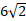​.

There are multiple ways of simplifying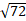​. Can you find the different methods of solving it? Try it!

Once you’ve done, take a look at this one.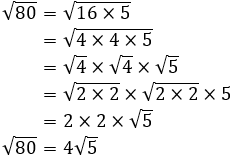The problem is similar to the one before. The only difference is that we split apart the 4s into two 2s for each, giving it more explanations as to how the radical expressions with the radicands of 4 are simplified.

### The Quotient Rule of Radical Expressions

The Quotient Rule denotes the property of radicals differently. That is, the division between two or more radical expressions with the same index—let’s say n—is always equal to the radical expression of the quotient between the previous radicands, with the index of n. Look at the following: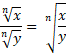Well, looking simply at the definition will hardly do any good. Let’s sharpen our calculation skill with a couple of examples: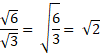Voila! The rather convoluted expression of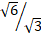​ is easily solved using the rule.

Let’s take a look at a more difficult problem: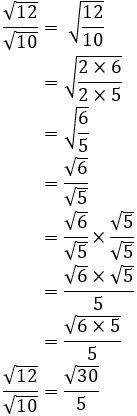This time, the road we took is longer but it’s actually not that different. Look at the process and you’ll see that it only takes simple calculations.

In case you are wondering, we multiply the equation with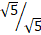​ to rationalize the denominator​ equals 1, so the figure won’t change the underlying value of the equation if multiplied with it.

Be the first to comment below.

### Comment Preview

HTML: You can use simple tags like <b>, <a href="...">, etc.

To enter math, you can can either:

1. Use simple calculator-like input in the following format (surround your math in backticks, or qq on tablet or phone):
a^2 = sqrt(b^2 + c^2)
(See more on ASCIIMath syntax); or
2. Use simple LaTeX in the following format. Surround your math with $$ and $$.
$$\int g dx = \sqrt{\frac{a}{b}}$$
(This is standard simple LaTeX.)

NOTE: You can mix both types of math entry in your comment.